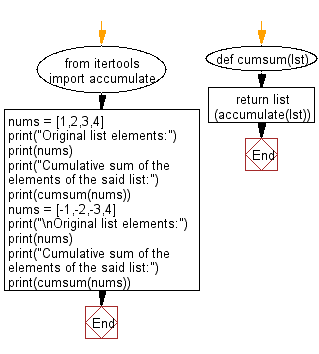﻿ Python: Cumulative sum of the elements of a given list - w3resource# Python: Cumulative sum of the elements of a given list

## Python List: Exercise - 267 with Solution

Write a Python program to get the cumulative sum of the elements of a given list.

Sample Solution:

Python Code:

``````from itertools import accumulate
def cumsum(lst):
return list(accumulate(lst))
nums = [1,2,3,4]
print("Original list elements:")
print(nums)
print("Cumulative sum of the elements of the said list:")
print(cumsum(nums))
nums = [-1,-2,-3,4]
print("\nOriginal list elements:")
print(nums)
print("Cumulative sum of the elements of the said list:")
print(cumsum(nums))
```
```

Sample Output:

```Original list elements:
[1, 2, 3, 4]
Cumulative sum of the elements of the said list:
[1, 3, 6, 10]

Original list elements:
[-1, -2, -3, 4]
Cumulative sum of the elements of the said list:
[-1, -3, -6, -2]
```

Flowchart:## Visualize Python code execution:

The following tool visualize what the computer is doing step-by-step as it executes the said program:

Python Code Editor:

Have another way to solve this solution? Contribute your code (and comments) through Disqus.

What is the difficulty level of this exercise?

Test your Python skills with w3resource's quiz

﻿

## Python: Tips of the Day

Floor Division:

When we speak of division we normally mean (/) float division operator, this will give a precise result in float format with decimals.

For a rounded integer result there is (//) floor division operator in Python. Floor division will only give integer results that are round numbers.

```print(1000 // 300)
print(1000 / 300)```

Output:

```3
3.3333333333333335```